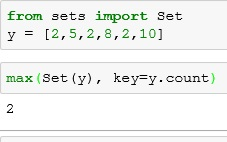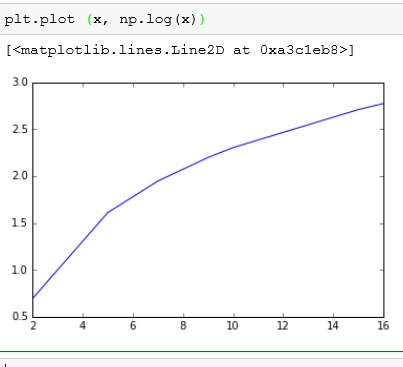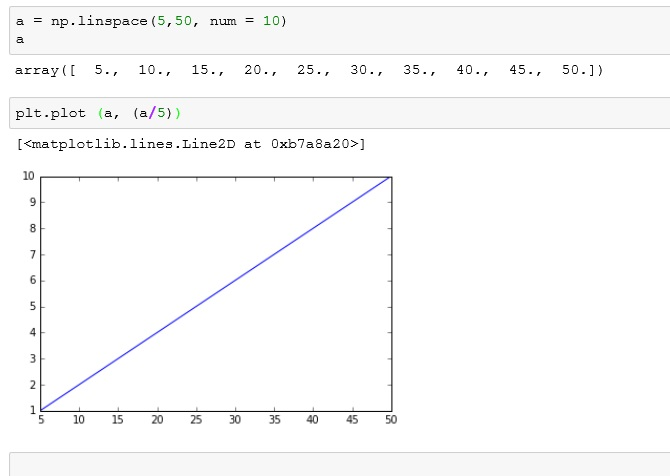# Python: Numpy Part II

Beyond numpy’s usefulness in creating arrays and matrices, numpy also provides a great suite of math functions that – for anyone with any programming background – are fairly intuitive.

Here are some examples:

np.pi returns pi and np.sqrt() takes the square root of whatever value you feed it.### Trig Functions

numpy handles most common trigonometry functions.### Stats

Numpy handles many statistics functions.

Below we have mean and median. Unfortunately, just like in R, there is no mode command, but we can fake it using Set.using set to fake modeNumpy can also be used to find range, variance, and standard deviation.### Rounding

Numpy has rounding features for dealing with decimals. It also has floor() and ceil() functions that bring the number down to the “floor” or up to the “ceiling”## Use in creating graphs

use np.sin()use np.log()you can even put the two together### linspace()

My final one today is a function called linspace(). It lets you create a start and finish point, and how many elements you want. It then will create a even list of number between start and finish.

linspace(start,finish, num=numbers you want)If you enjoyed this lesson, click LIKE below, or even better, leave me a COMMENT.

Last Lesson: Numpy

Next Lesson: Pandas (Series)